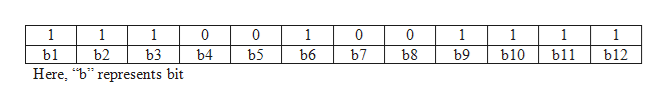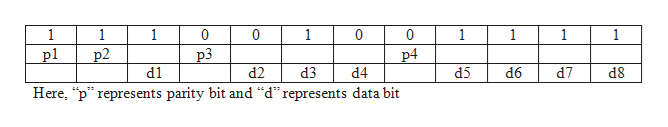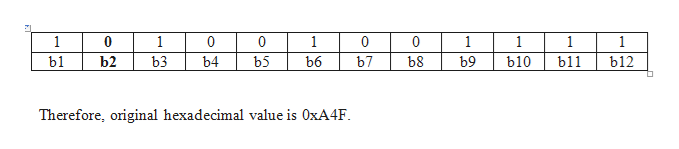# A 12-bit (8,4) Hamming code whose hexadecimal value is 0xE4F arrives at a receiver.What was the original value in hexadecimal?Assume that not more than 1 bit is in error.

Question
189 views

12-bit (8,4) Hamming code whose hexadecimal value is 0xE4F arrives at a receiver.

What was the original value in hexadecimal?

Assume that not more than 1 bit is in error.

check_circle

Step 1

0xE4F = 111001001111help_outlineImage Transcriptionclose1 1 1 0 0 1 0 1 1 1 1 bl b2 ь3 b4 b5 ь6 b7 ь8 ь9 b10 bl1 b12 Here, "b represents bit fullscreen
Step 2

Split the bits into parity bits and data bits as follows:help_outlineImage Transcriptionclose1 1 1 1 0 0 0 1 1 1 1 pl р2 р3 р4 d1 d2 d3 d4 d5 d6 d7 d8 Here, "p" represents parity bit and "d"represents data bit fullscreen
Step 3

p1 = b3 + b5 + b7 + b9 + b11

= 1 + 0 + 0 + 1 + 1

= 1

p1 value is same bit value as in the table

p2 = b3 + b6 + b7 + b10 + b11

= 1 + 1 + 0 + 1 + 1

= 0

p2 value is not same bit value as in the table

p3 = b5 + b6 + b7 + b12

&n...help_outlineImage Transcriptionclose1 0 1 0 0 1 0 0 1 1 1 1 bl1 b10 b12 b1 b2 ьз b4 b5 ь6 b7 ь8 ь9 Therefore, original hexadecimal value is OXA4F fullscreen

### Want to see the full answer?

See Solution

#### Want to see this answer and more?

Solutions are written by subject experts who are available 24/7. Questions are typically answered within 1 hour.*

See Solution
*Response times may vary by subject and question.
Tagged in

### Computer Science